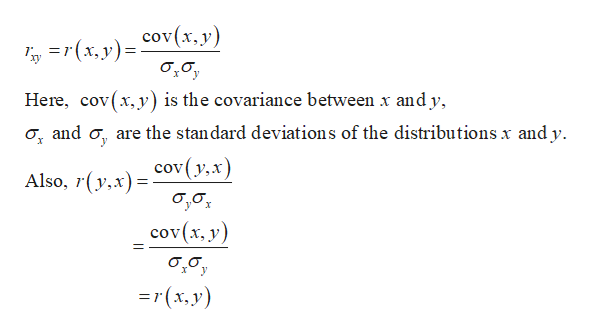In the formula for r, if we exchange the symbols x and y, do we get a different result or do we get the same (equivalent) result? Explain your answer.The result is different because the formula is not dependent on the symbols.The result is the same because the formula is not dependent on the symbols.    The result is different because the formula is dependent on the symbols.The result is the same because the formula is dependent on the symbols.(b) If we have a set of x and y data values and we exchange corresponding x and yvalues to get a new data set, should the sample correlation coefficient be the same for both sets of data? Explain your answer.The result is different because the formula is not dependent on which values are the x values and which values are the y values.The result is the same because the formula is not dependent on which values are the x values and which values are the y values.    The result is different because the formula is dependent on which values are the x values and which values are the y values.The result is the same because the formula is dependent on which values are the x values and which values are the y values.(c) Compute the sample correlation coefficient r for each of the following data sets and show that r is the same for both. (Use 3 decimal places.)

Question

In the formula for r, if we exchange the symbols x and y, do we get a different result or do we get the same (equivalent) result? Explain your answer.

The result is different because the formula is not dependent on the symbols.The result is the same because the formula is not dependent on the symbols.    The result is different because the formula is dependent on the symbols.The result is the same because the formula is dependent on the symbols.

(b) If we have a set of x and y data values and we exchange corresponding x and yvalues to get a new data set, should the sample correlation coefficient be the same for both sets of data? Explain your answer.

The result is different because the formula is not dependent on which values are the x values and which values are the y values.The result is the same because the formula is not dependent on which values are the x values and which values are the y values.    The result is different because the formula is dependent on which values are the x values and which values are the y values.The result is the same because the formula is dependent on which values are the x values and which values are the y values.

(c) Compute the sample correlation coefficient r for each of the following data sets and show that r is the same for both. (Use 3 decimal places.)

Step 1

Note:

Hi there! Thank you for posting the question! However, we are unable to answer the question in Part (c) since you have not posted the data set for computing the sample correlation coefficient. If you want the answer for Part (c), please re-post the question with the data set.

Step 2

Correlation coefficient - r:

The Karl Pearson’s product-moment correlation coefficient or simply, the Pearson’s correlation coefficient is a measure of the strength of a linear association between two variables and is denoted by r or rxy.

The coefficient of correlation rxy between two variables x and y for the bivariate data set (xi, yi) for i=1,2,3…N is given below:help_outlineImage Transcriptionclosex,yCov(x, y) Here, cov(x,y) is the covariance between x andy o, and o are the standard deviations of the distributions x and y Also, (y,xcov(y,x) cov(х, у) 37(х, у) fullscreen
Step 3

The Pearson’s product-moment correlation does not take into consideration whether a variable has been classified as a dependent or independent variable. It treats all variables equally. A change of scale and origin does not affect the value of r. Indeed, the...

Want to see the full answer?

See Solution

Want to see this answer and more?

Our solutions are written by experts, many with advanced degrees, and available 24/7

See Solution
Tagged in

Other